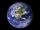# Tropical, mild and arctic

How many percents of the Earth's surface lies in the tropical, mild and arctic range? The border between the ranges is the parallel 23°27 'and 66°33'.

Result

p1 =  8.259 %
p2 =  51.946 %
p3 =  39.795 %

#### Solution:Leave us a comment of example and its solution (i.e. if it is still somewhat unclear...):

Showing 0 comments:Be the first to comment!#### To solve this example are needed these knowledge from mathematics:

Our percentage calculator will help you quickly calculate various typical tasks with percentages.

## Next similar examples:

1. PersonsPersons surveyed:100 with result: Volleyball=15% Baseball=9% Sepak Takraw=8% Pingpong=8% Basketball=60% Find the average how many like Basketball and Volleyball. Please show your solution.
2. The ballThe ball was discounted by 10 percent and then again by 30 percent. How many percent of the original price is now?
3. Hemispherical hollowThe vessel hemispherical hollow is filled with water to a height of 10 cm =. How many liters of water are inside if the inside diameter of the hollow is d = 28cm?
4. Three workshopsThere are 2743 people working in three workshops. In the second workshop works 140 people more than in the first and in third works 4.2 times more than the second one. How many people work in each workshop?
5. TeamsHow many ways can divide 16 players into two teams of 8 member?
6. PIN - codesHow many five-digit PIN - code can we create using the even numbers?
7. Earth's surfaceThe greater part of the earth's surface (r = 6371 km) is covered by oceans; their area is approximately 71% of the Earth's surface. What is the approximate area of the land?
8. Fit ballWhat is the size of the surface of Gymball (FIT - ball) with a diameter of 65 cm?
9. Profit gainIf 5% more is gained by selling an article for Rs. 350 than by selling it for Rs. 340, the cost of the article is:
10. BorrowingI borrow 25,000 to 6.9% p.a.. I pay 500 per month. How much will I pay and for how long?
11. Percentage 1052 shorts and missed 13. Calculate percentage
12. Theorem proveWe want to prove the sentense: If the natural number n is divisible by six, then n is divisible by three. From what assumption we started?
13. Reference angleFind the reference angle of each angle:
14. AP - simpleDetermine the first nine elements of sequence if a10 = -1 and d = 4
15. TrigonometryIs true equality? ?
16. Elimination methodSolve system of linear equations by elimination method: 5/2x + 3/5y= 4/15 1/2x + 2/5y= 2/15
17. LegsCancer has 5 pairs of legs. The insect has 6 legs. 60 animals have a total of 500 legs. How much more are cancers than insects?